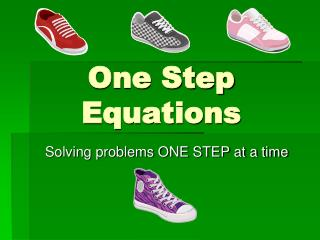# One Step Equations - PowerPoint PPT PresentationDownload PresentationOne Step Equations

One Step EquationsDownload Presentation## One Step Equations

- - - - - - - - - - - - - - - - - - - - - - - - - - - E N D - - - - - - - - - - - - - - - - - - - - - - - - - - -
##### Presentation Transcript

1. One Step Equations Solving problems ONE STEP at a time

2. One Step Equations What am I Learning Today? How will I show that I learned it? Use order of operations to solve mathematical equations Write and evaluate algebraic expressions, including those with exponents Solve simple one step equations using the four basic operations and rational numbers

3. Vocabulary • Equation: A mathematical sentence that contains an equal sign • Variable: A letter or symbol used to represent a number • Algebraic Expression: A mathematical phrase involving one or more variables and operation symbols • Evaluate: Perform operations to obtain a single value for a numerical or algebraic expression • Order of Operations: The rules that must be followed when simplifying expressions • Exponent: Tells how many times the base is used as a factor

4. Who is right? • How did the boy solve this problem? • How did the girl solve this problem? When performing arithmetic operations there can be only one correct answer. We need a set of rules in order to avoid this kind of confusion. Mathematicians have devised a standard order of operations for calculations involving more than one arithmetic operation.

5. Questions Notes Questions Answers • - Parentheses: Simplify the inside of all parentheses first • Exponents: Simplify the exponent of every number • - Multiplication and Division: Simplify one at a time • - Addition and Subtraction: Simplify one at a time What are the Order of Operations? How can I remember the Order of Operations? Please Excuse My Dear Aunt Sally (PEMDAS) What if there are several multiplication and division or addition and subtraction operations? ** Always keep things in order - Solve operations as they appear from left to right- Multiplication DOES NOT have to be solved before division - Addition DOES NOT have to be solved before subtraction **Rewrite the equation after solving each part from left to right

6. Exponent Base Let’s Talk about Exponents • A number is in exponential form when it is written with a base and an exponent. 73 = 7  7  7= 343 Find the value. 26 = 2  2  2  2  2  2 4 x 2 x 2 x 2 x 2 8 x 2 x 2 x 2 16 x 2 x 2 32 x 2 = 64

7. Let’s Talk about Parentheses • Parentheses ( ) or brackets [ ] or { } are like a cocoon…what is inside must be complete before it can leave the cocoon. • Order of operations MUST be used inside parentheses or brackets if there is more than one operation being used. • Always complete the operations in the parentheses or brackets the furthest inside • For Example: {[24 ÷ (2 x 3)] + 9} – 7 = ?

8. Perform operations within parentheses. Multiply. Add. Find the value of the number with the exponent. Multiply. Add. Order of Operations: PEMDAS Evaluate the expressions. [7 + (24 ÷ 6)]  3 42 + (3  7) [7 + 4]  3 11 x 12 132 42 + (21) 16 + 21 37

9. [24 ÷ (9 – 6)]  (33 – 10)   (33 – 10) [24 ÷ 3]  (33 – 10) Perform operations within brackets and parentheses. Multiply. Perform operation in parentheses. Find the value of the number with the exponent. Now Try This! Evaluate the expression. [24 ÷ (9 – 6)]  (33 – 10) 8  (27 – 10) 8 x 17 136

10. Order of Operation Practice 1. 35 ÷ 7 – 5 = 2. 80 x 2 ÷ 40 – 1= 3. 2 + 2² x (5 + 4) ÷ (2³ + 4) = 4. 8 + (2 x 5) x 18 ÷ 9= 5. 12 x [2² x(4 • 3)] ÷ 6 – 9 = 0 3 5 28 87

11. Dice Substitution • Find a partner. • Designate each color dice #1 and #2 • Roll dice #1. Substitute the value for the variable and evaluate. • Roll dice #2. Substitute the value for the variable and evaluate. • Find the sum of the determined values. This is your score. • The person with the highest score wins!

12. Paired Discussion Turn to someone around you and discuss the following: Why do you have to use the order of operations to evaluate each expression? When performing arithmetic operations there can be only one correct answer. We need a set of rules in order to avoid confusion and help us evaluate the expression in the same way each time. What would happen if there were no order of operations rules? People might perform operations in different orders and arrive at different solutions. The purpose of the order of operations is to guarantee that every numerical expression has a unique value.